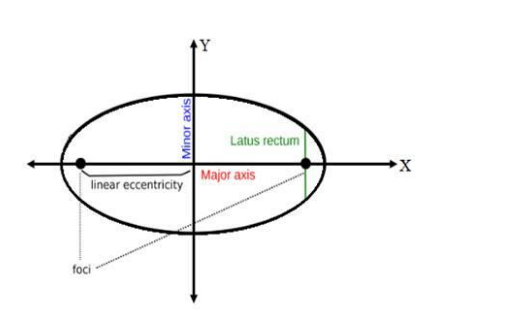# Find the eccentricity of an ellipse whose latus rectum is one half of its major axis.`
Question:

Find the eccentricity of an ellipse whose latus rectum is one half of its major axis.

Solution:Let the equation of the required ellipse is

$\frac{x^{2}}{a^{2}}+\frac{y^{2}}{b^{2}}=1$  …(i)

It is given that,

Length of Latus Rectum $=\frac{1}{2}$ major Axis

We know that,

Length of Latus Rectum $=\frac{2 b^{2}}{a}$

and Length of Minor Axis $=2 \mathrm{a}$

So, according to the given condition,

$\frac{2 b^{2}}{a}=\frac{1}{2} \times 2 a$

$\Rightarrow \frac{2 b^{2}}{a}=a$

$\Rightarrow 2 b^{2}=a^{2} \ldots(i i)$

$\Rightarrow \mathrm{a}=\sqrt{2 \mathrm{~b}^{2}}$

$\Rightarrow \mathrm{a}=\mathrm{b} \sqrt{2}$

Now, we have to find the eccentricity

We know that,

Eccentricity, e $=\frac{c}{a}$ …(iii)

where, $c^{2}=a^{2}-b^{2}$

So, $c^{2}=2 b^{2}-b^{2}[$ from (ii) $]$

$\Rightarrow c^{2}=b^{2}$

$\Rightarrow c=\sqrt{b^{2}}$

$\Rightarrow c=b$

Substituting the value of c and a in eq. (iii), we get

Eccentricity, $e=\frac{c}{a}$

$=\frac{b}{b \sqrt{2}}$

$\therefore \mathrm{e}=\frac{1}{\sqrt{2}}$